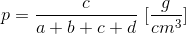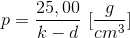## Foundry sand pycnometer

Device to determine the density of foundry mold material or other similar substances (Fig. 1). To determine the density, weighing is applied to determine:

a = weight of the empty pyknometer including plug,
b = weight of the pyknometer filled with water including plug,
c = weight of the sand sample (exactly 25.00 g) and
d = weight of the pyknometer including sand sample and fully filled with water.

Respectively, the density of the sand can be calculated according to Eq. 1:

Eq. 1As the sum of k = (a + b + c) is constant for the same pyknometer with a sand sample of 25.00 g, the following applies:

Eq. 2For this reason, it is necessary to determine the constant value k of the pyknometer to determine the sanddensity as follows: The pyknometer is filled with water (1/3 water) and the sand sample to be tested is inserted. Anti-foaming agent is applied to ensure discharge of the air bubbles. Afterwards, the pyknometer is completely filled with water and closed with the plug. The total weight d is determined by weighing and the sanddensity is calculated according to Eq 2.

The described process only applies for humid molds sand.